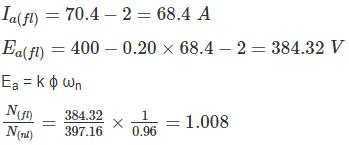Courses

# Test: Electrical Machines- 4

## 10 Questions MCQ Test GATE Electrical Engineering (EE) 2022 Mock Test Series | Test: Electrical Machines- 4

Description
This mock test of Test: Electrical Machines- 4 for Electrical Engineering (EE) helps you for every Electrical Engineering (EE) entrance exam. This contains 10 Multiple Choice Questions for Electrical Engineering (EE) Test: Electrical Machines- 4 (mcq) to study with solutions a complete question bank. The solved questions answers in this Test: Electrical Machines- 4 quiz give you a good mix of easy questions and tough questions. Electrical Engineering (EE) students definitely take this Test: Electrical Machines- 4 exercise for a better result in the exam. You can find other Test: Electrical Machines- 4 extra questions, long questions & short questions for Electrical Engineering (EE) on EduRev as well by searching above.
QUESTION: 1

### A short shunt compound wound motor is having series field resistance, armature resistance and shunt field resistance of 0.6 Ω, 0.4 Ω and 250 Ω respectively. If the motor is drawing 50 A at 500 V, what the voltage drop across series field resistance?

Solution:

Short shunt motor be like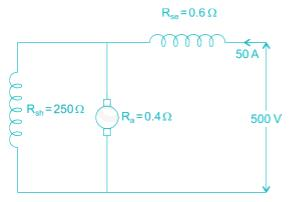Voltage drop across series field resistance
= 50 × 0.6
= 30 V

QUESTION: 2

### A DC shunt motor of 200 V, 10.5 A, 2000 rpm has an armature resistance of 0.5 Ω and field resistance of 400 Ω. It drives a load whose torque is constant at rated motor torque. What is the value of armature current if the source voltage drops to 175 V?

Solution:

Given V1 = 200 V
Armature resistance (Ra) = 0.5 Ω
Field winding resistance (Rsh) = 400 Ω
Field current = 200/400 = 0.5 A
Load current = 10.5 A
Armature current = 10.5 – 0.5 = 10 A
Given that load torque is constant.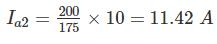*Answer can only contain numeric values
QUESTION: 3

### A six pole, 60 kW DC machine operating at 1200 rpm has a generated emf of 150 V. If the speed is reduced to 70% of its original value and the pole flux is tripled, then induced emf would be___ (in V)

Solution:

In a DC machine,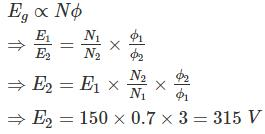QUESTION: 4

A long shunt compound wound motor is having shunt field resistance, series field resistance and armature resistance of 300 Ω, 0.8 Ω and 0.6 Ω respectively. If the motor is drawings 60 A at 500 V, what is the voltage drop across series field resistance?

Solution:

Long shunt motor circuit is given as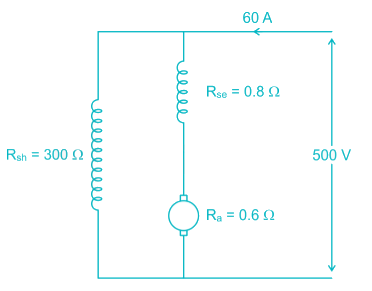Current through shunt field = 500/300 = 1.67 A
∴ Armature current Ia = 58.33A
Voltage across series field resistance
= 58.33 × 0.8
= 46.664 V

*Answer can only contain numeric values
QUESTION: 5

A 220 V DC shunt motor takes 3 A at no-load. It draws 25 A when running at full-load at 1500 rpm. The armature and shunt resistances are 0.5 Ω and 220 Ω, respectively. The no- load speed in rpm (round off to two decimal places) is ________.

Solution:

Voltage (V) = 220 V
No load current (INL) = 3 A
Full load current (IFL) = 25 A
Full load speed (NFL) = 1500 rpm
Armature resistance (Ra) = 0.5 Ω
Shunt resistance (Rsh) = 220 Ω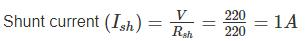Armature current (Ia) = IL - Ish
When motor is operating at no load
IanL = INL - Ish = 3 – 1 = 2 A
Eb1 = V - IanL Ra
= 220 – (2) (0.5) = 219 V
When motor is operating at full load
IaFL = IFL - Ish = 25 – 1 = 24 A
Eb2 = V – IaFL Ra
= 220 – (24) (0.5) = 208 V
We know that, in a DC shunt motor
Eb α N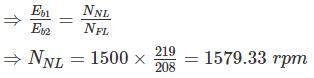*Answer can only contain numeric values
QUESTION: 6

A 600 V series motor of resistance 1 Ω runs at 800 rpm when the current is 60 A. If an identical motor is mechanically coupled and joined in series, what will be the current (in A) when the set runs at 400 rpm and exerts twice the original gross torque?

Solution:

Eb1 = V − IaRa = 600 − 1 × 60 = 540
Let the current when joined in in series = Ia2
The resistance = 2 × 1 = 2 Ω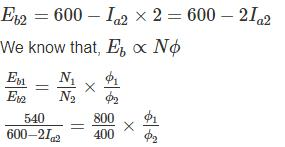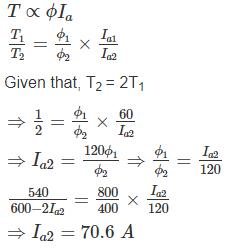*Answer can only contain numeric values
QUESTION: 7

A 250-V DC shunt motor has a shunt field resistance of 150 Ω and an armature resistance of 0.3 Ω. For a given load. The motor runs at 1400 rpm, and drawing 20 A current. If a resistance of 75 Ω is added in series with the field, find the new armature current (in A). Assume load torque and flux and constant.

Solution:

The field current corresponds to: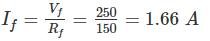Now:
IA = IL - If = 20 – 1.66 = 18.34 Amps
Observe that the current of the field circuit is much smaller than the armature current. Thus, adding the speed control resistance leads to minor power dissipation.

Ea = VS – IaRa = 250 – 5.502 = 244.5 V

EA = K ϕ ω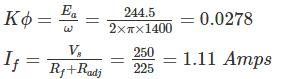The new armature current corresponds to:
Ia = I– If = 20 – 1.11 = 18.89 Amps

*Answer can only contain numeric values
QUESTION: 8

A separately excited DC generator, when running at 1200 rpm supplies 200 A at 125 V to a circuit of constant resistant what will be the current (in amp) when the speed is dropped to 1000 rpm and the field is reduced to 80%. Armature resistance is 0.04 Ω and total drop at brushes is 2V.

Solution:

Eg1 = VL + IaRa + 2 = 125 + (0.04 × 200) + 2 = 135 V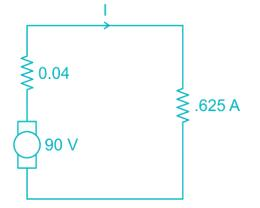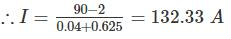*Answer can only contain numeric values
QUESTION: 9

A 440 V DC shunt motor is running at 1000 RPM at full load torque, reduced armature voltage and full field. If load torque is reduced to 50% of rated value with armature voltage and field voltage held constant at previous value, the speed increase 1050 rpm. Find armature voltage drop (in V) at full load. Neglect effect of armature reaction. [Take no load speed 1000 rpm]

Solution:

Ea1 = (440 – Ia1ra)
Ea1 = k ϕ1 ω1
Motor torque T1 = kaϕ1Ia1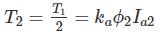As field voltage held constant ϕ2 = ϕ1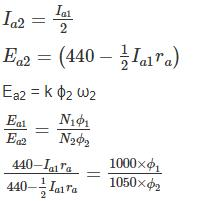440 × 21 – 21 Ia1r = 440 × 20 – 10 Ia1ra

∴ Armature voltage drop Ia1ra = 440/11 = 40 V

*Answer can only contain numeric values
QUESTION: 10

A 400 V dc shunt motor takes a current of 6.2 A on no load and 70.4 A on full - load. Armature reaction weakens the field by 4%. Calculate the ratio of full-load speed to no-load speed. Given Ra = 0.20 Ω, Brush voltage drop = 2 V, Rf = 200 Ω.

Solution:

Field current If  = 400/200 = 2 A
No-load
Iao = 6.2 – 2 = 4.2 A
Eao = 400 – 0.20 × 4.2 – 2 = 397.16 V
Full load: